# How to Count Cells With Text in Excel

•Android
•iPhone
•Mac
•Windows
• Device Missing?

Excel spreadsheets are a great way to store and analyze data. Spreadsheets usually get made up of cells with a combination of numbers and text. To understand your data more, you need to differentiate the cells with text. This article explains how you can select a cell range then use the “COUNTIF” function to count the cells containing text. In addition, you can learn how to find specific, duplicate, and colored text in your spreadsheet.## How to Count Cells With Text in Excel on a Windows PC

To count the cells that contain text within your spreadsheet on a Windows computer, do the following:

1. Click on an “empty cell” on your spreadsheet to insert the formula.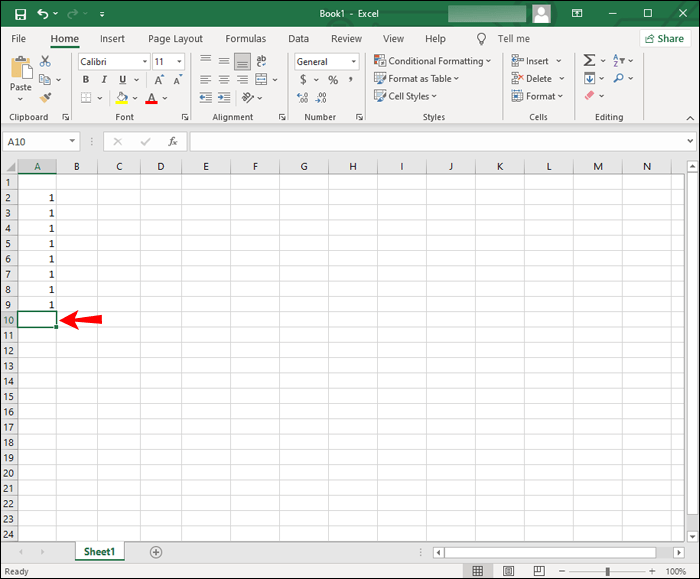2. Type or paste the function `=COUNTIF (range, criteria)` without quotes to count the number of cells containing text within a specific cell range.3. For “range,” enter the cell range you want to check. Type the first and last cells separated by a colon. For example, to count cells A2 to A9 you’d enter “`A2:A9`.”4. For “criteria,” type “`"*"`” with quotes. This counts the number of cells that cointain text within the specified range. The complete formula should look similar to “`=COUNTIF (A2:A9, “*”)`.”5. Now, press “enter” to apply the formula. The result will display in the formula’s cell.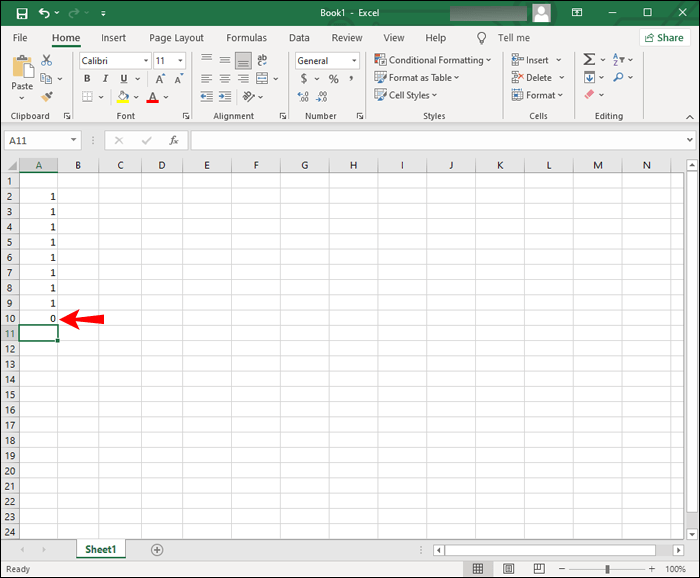## How to Count Cells With Text in Excel on a Mac

The steps for counting the cells that have text within your spreadsheet via macOS are the same as with Excel for Windows:

1. Launch “Excel,” then open the spreadsheet you want to analyze. You can also just “double-click” the file if it has Excel as its default program.
2. Select an “empty cell” on your spreadsheet to enter the formula.
3. In the empty cell, type “`=COUNTIF (range, criteria)`.” This formula counts the number of cells in the specified range with text in them.
4. For “range,” type the cell range you want to look at. Enter the first and last cells, divided by a colon. For example, to count cells B2 to B10 you’d enter “`B2:B10`.”
5. For the “criteria” part, type “`“*”`” with quotes. This counts the number of cells with text in the range you typed. For example, your complete formula should look similar to “`=COUNTIF (B2:B10, “*”)`.”
6. On your keyboard, press “enter” to apply the formula. The result appears in the selected cell.

## How to Count Cells With Text in Excel 365

To count the cells containing text in your spreadsheet using Excel 365, you apply the same “COUNTIF” function used in Excel for Windows and macOS. Here’s how:

1. Open the “Excel spreadsheet” you wish to examine.
2. Click on an “empty cell” to type the formula.
3. In the empty cell, type: “`=COUNTIF (range, criteria)`.” This formula counts the number of cells with text in them from within your specified cell range.
4. For the “range” part, type the cell range you want to look at. Enter the first and last cells separated by a colon. For example, to count cells C2 to C11 enter “`C2:C1`.”
5. For “criteria,” type or paste “`“*”`” with quotes. This counts the number of cells (in your spedified range) that have text in them. For example, your complete formula should look something like “`=COUNTIF (C2:C11, “*”)`.”
6. Now press “enter” to apply the formula you just created. The result will display in the formula’s cell.

## How to Count Cells With Text in Excel on the iPhone App

To count the number of cells containing text within your spreadsheet using the Excel app on an iPhone, do the following:

1. Launch the “iPhone Excel App.”
2. Tap on “Open” to view your saved spreadsheets, then select the specific “Excel file” to open it.
3. “Double-tap” on an “empty cell” in the spreadsheet to enter the “COUNTIF” formula, or you can “long-press” an “empty cell” then tap “Edit” from the pop-up menu.4. In the empty cell, type “`=COUNTIF (range, criteria)`.” This formula counts the number of cells containing text inside your cell range.5. For the “range” part, type the “cell range” you want to count. Enter the first and last cells separated by a colon. To count cells D2 to D12 enter “`D2:D12`”.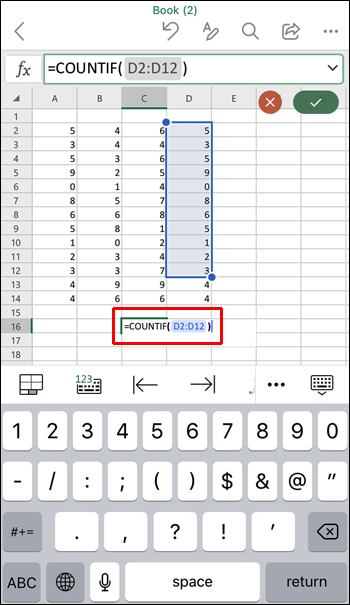6. For the “criteria,” type “`“*”`” with quotes. This counts the number of cells with text in the range. The complete formula should look something like: “`=COUNTIF (D2:D12, “*”)`.”7. Now, tap “enter” to apply the created formula. The result will appear in the formula’s cell.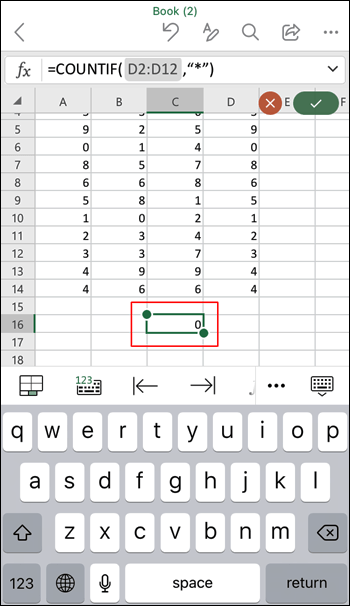## How to Count Cells With Text in Excel on the Android App

To count the number of cells that have text in your spreadsheet using the Android Excel app, do the following:

1. Launch the “Android Excel app.”
2. Open the spreadsheet you want to examine by tapping on “Open” to see your saved spreadsheets, then tap on the “desired file” to automatically open it.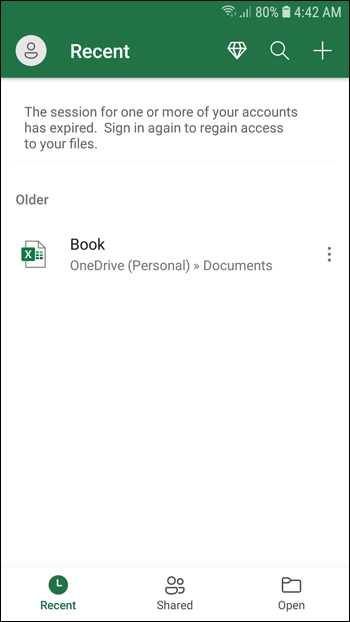3. “Double-tap” on an “empty cell” to enter the “COUNTIF” formula. Alternatively, “long-press” an “empty cell,” then tap “Edit” from the pop-up menu.4. In the empty cell, enter “`=COUNTIF (range, criteria)`” without quotes. This formula counts the number of cells with text inside the cell range.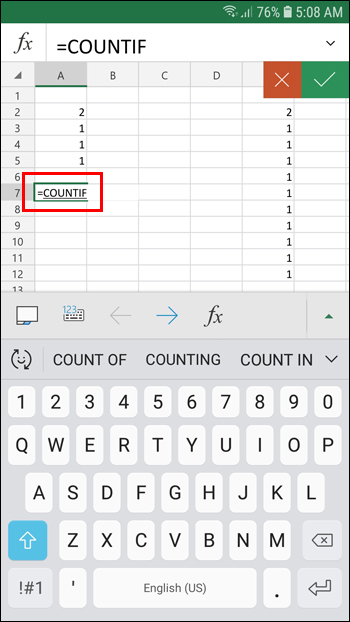5. For the “range” part of the formula, enter the cell range you wish to count. Enter the first and last cells separated by a colon. To count cells E2 to E12 from one column, enter “`E2:E12`.”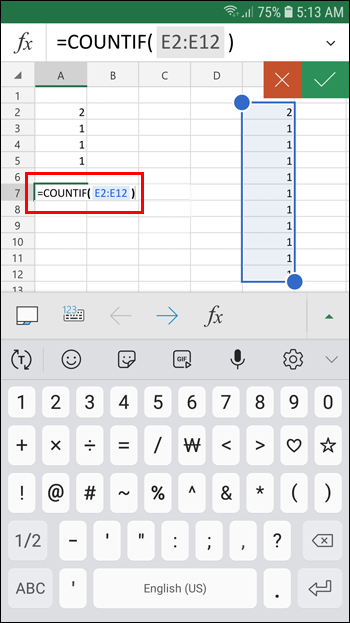6. For the “criteria” part of the formula, type “`“*”`” with quotes. This counts the number of cells with text in the specified range, including more than one row. Your complete formula should look something like: “`=COUNTIF (A2:E12, “*”)`.”7. Now tap “enter” to apply the formula. The result displays in the formula’s cell.## How to Count Cells in Excel That Have Specific Text

By using the “COUNTIF” function, you can count the number of cells that contain specific text strings, such as “Excel,” “John,” or “John Meyers.” The formula is similar to counting cells that have any kind of text in them, but you change the “criteria” part of the formula to search for specific text. In this example, you’ll see the number of times the word “Excel” appears in a specific cell range:

1. Launch “Excel” on your device and open the “designated file,” or just launch the “designated file” if it is set to open Excel by defualt.
2. Click on an “empty cell” to type the formula.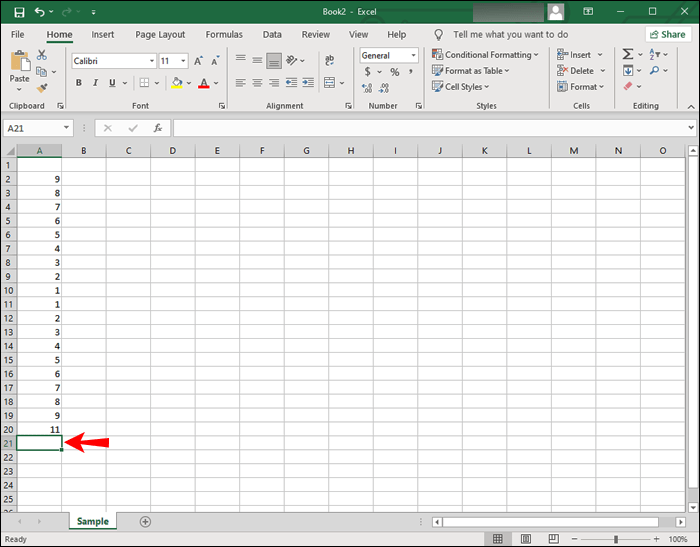3. In the empty cell, type “`=COUNTIF (range, criteria)`” without quotes.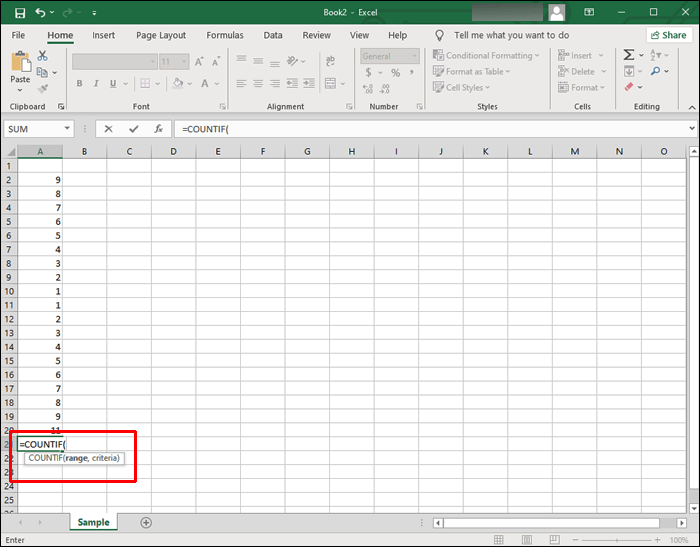4. For the “range” part of the formula, enter the cell range you wish to count. Enter the first and last cells separated by a colon. To count cells A2 to A20 enter “`A2:A20`” without quotes.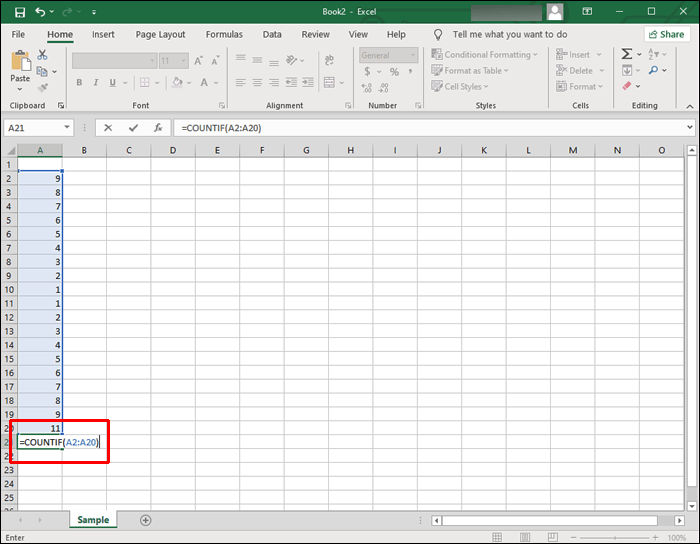5. For the “criteria” section of the formula, type ” `"Excel"` ” with quotes. This counts the number of cells with “Excel” in the specified range. Your formula should look something similar to “`=COUNTIF (A2:A20, “Excel”)`.”## How to Count Cells With Duplicate Text in Excel

Besides counting cells with text and specific text, you can count the number of cells with duplicate content.

In the following example, we’re looking for duplicate student grades. Our spreadsheet is set up as follows:

• Column A – lists our students A2:A10
• Column B – lists each student’s grades (A, B, or C)
• Column D –lists the available grades. D2 for “As,” D3 for “Bs,” and D4 for “Cs.”
• Column E – lists the count of each grade.

### Count Cells With Duplicate Text Including First Instance

To count the number of cells in your spreadsheet with instances of grade “A,” “B,” or “C” – including the first instance – enter the following formulas:

• For instances of grade “A,” click cell E2 and type formula “`=COUNTIF (B2 : B10, D2)`”.• For instances of grade “B,” click cell E3 and type formula “`=COUNTIF (B2 : B10, D3)`”.• For instances of grade “C,” click cell E4 and type formula “`=COUNTIF (B2 : B10, D4)`”.Now you have the count for duplicate grades including the first instance listed in column “E.”

### Count Cells With Duplicate Text Excluding First Instance

To count the number of cells in your spreadsheet with instances of grade “A,” “B,” or “C” – excluding the first instance – enter the following formulas:

• For instances of grade “A” excluding the first instance, click cell E2 and type formula “`=COUNTIF (\$B\$2 : \$B\$10, D2)-1`”.• For instances of grade “B” excluding the first instance, click cell E3 and type formula “`=COUNTIF (\$B\$2 : \$B\$10, D3)-1”.`• For instances of grade “C” excluding the first instance, click cell E4 and type formula “`=COUNTIF (\$B\$2 : \$B\$10, D4)-1`”.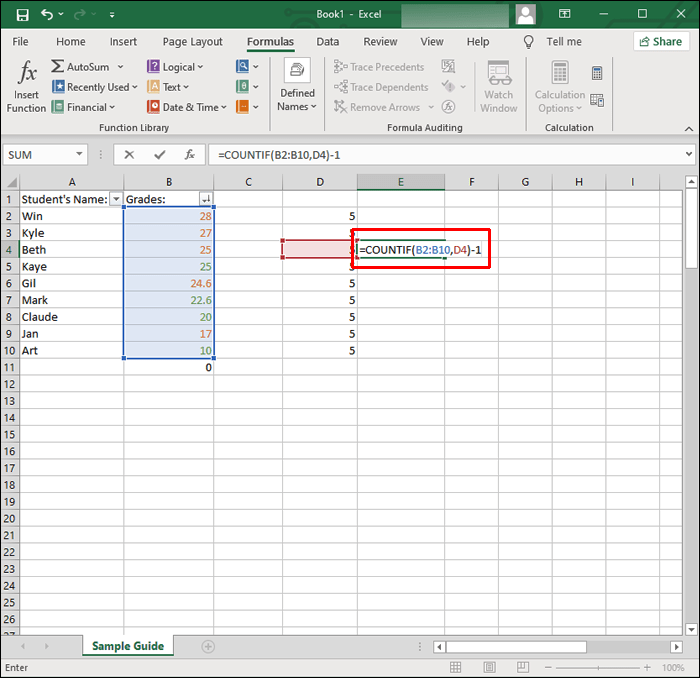Now you have the count for duplicate grades excluding the first instance listed in column “E.”

## How to Count Cells With Color Text in Excel

Excel does not have a formula to count cells based on their text color. To get around this, filter the results then count. Here’s how:

1. Open the spreadsheet you wish to analyze.2. Right-click a cell with the text of the color you wish to count.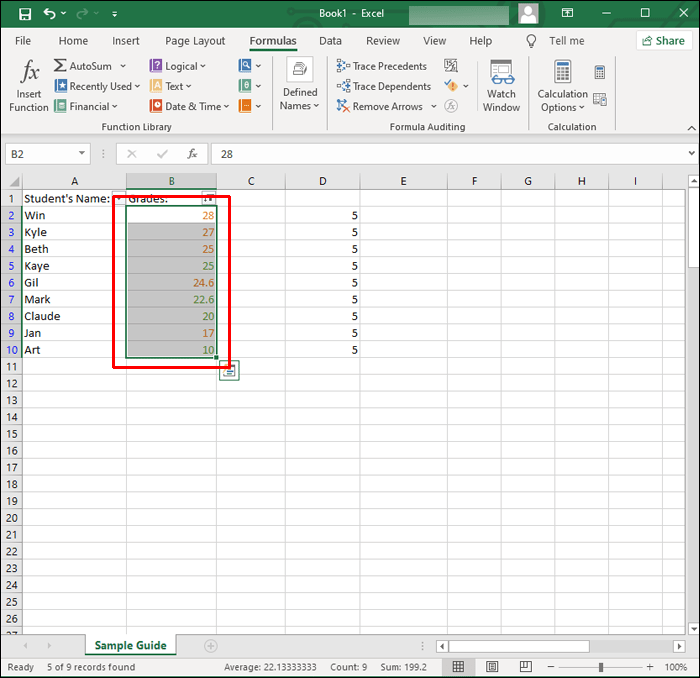3. Select “Filter,” then “Filter by Selected Cell’s Font Color” to filter the cells with the selected text color.4. Next, tell Excel to count your data range. If your text is listed from cell B2 to B20 enter the following formula: “`=SUBTOTAL (B2 : B20)`”.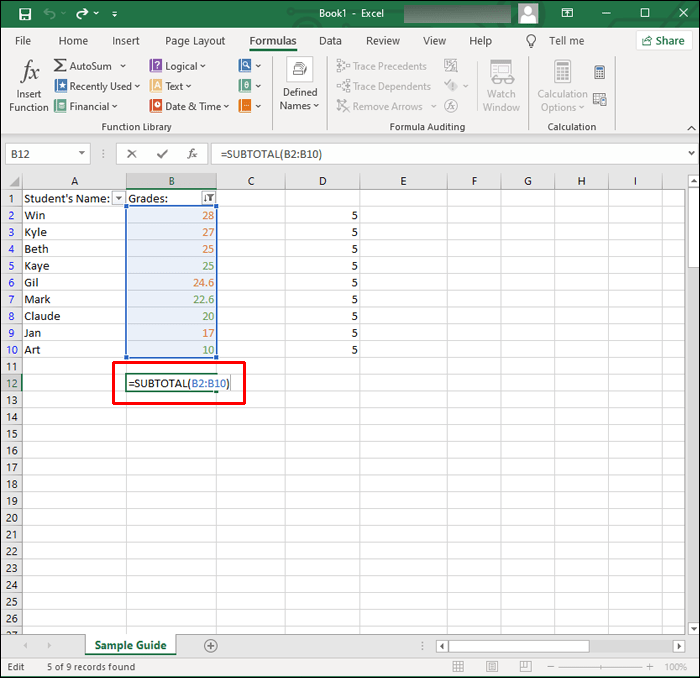Once you hit “Enter” to apply the filter, Excel will only display the cells containing that color and hide the remaining values.

The “SUBTOTAL” function will exclude the values in the hidden rows, therefore, returning the count for the selected text color only.

## Finding the Cells With Text

The Excel application does a great job of storing your data and facilitating analysis. It handles text as well as numbers.

Its over four hundred functions include the “COUNTIF” function. This function is useful for finding the total of cells containing specific information like cells with text, and the number of occurrences for specific text.

Were you able to find out what you needed to know about your spreadsheet data? How useful do you find Excel in general? Share your opinions in the comments section below.

Disclaimer: Some pages on this site may include an affiliate link. This does not effect our editorial in any way.

Todays Highlights